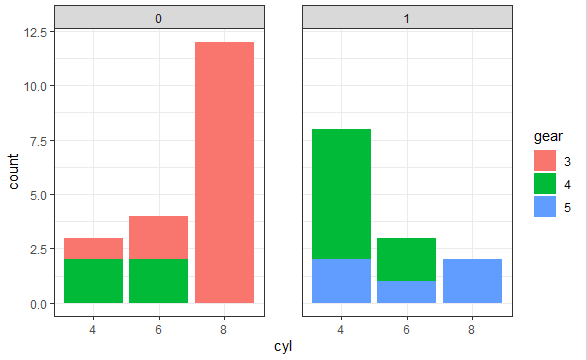2 views

I have several faceted histograms (obtained with the command below) which are nicely plotted one under the other. I would like to increase the spacing between them, however, they are tight.

I looked at the doc but didn't find a parameter for this.

qplot (Happiness.Level, Number.of.Answers, data=mydata, geom="histogram") + facet_grid (Location ~ .)

by

To increase the spacing between faceted plots, you can use the panel.spacing argument in the theme function as follows:

p + theme(panel.spacing = unit(2, "lines"))

For example:

library("ggplot2")

library(grid)

ggplot(mtcars, aes(x = cyl, fill = gear)) +

geom_bar()+

theme_bw()+

facet_grid(.~am)+

theme(panel.spacing = unit(2, "lines"))

Output: